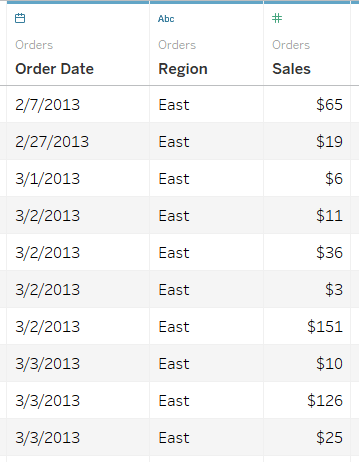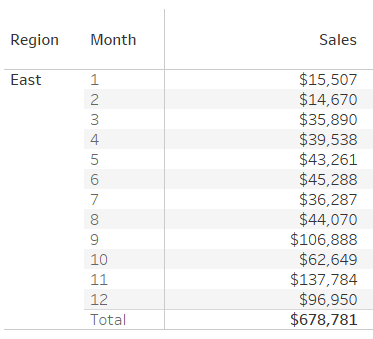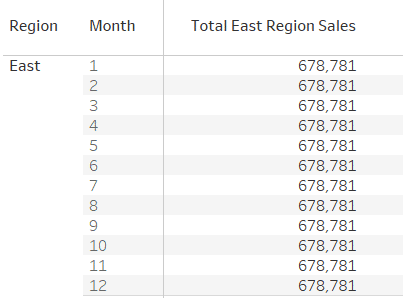# EXCLUDE – LOD Deep Dive in Tableau

EXCLUDE: “EXCLUDE” level of detail expression is used to omit specified dimensions from the aggregations. Using “EXCLUDE”, a user can omit the lower level granularity dimension which is present in the view and can directly calculate the value at a higher granularity level.

Tableau Training and Certification at Edureka“EXCLUDE” level of detail expression is majorly used to calculate ‘difference from overall average’ or ‘percent of total’

Example:

Requirement: Let’s consider the requirement is to compare total sales with the monthly sales of the East region.
Data: Consider the data with required “Order Date”, “Region” and “Sales” fields.Current view: To get the monthly sales we need to have “Region”, “Month” and “Sum of Sales” in the view. Therefore, in the current view if we directly use “SUM(Sales)” across “Months” then it will show the total value of sales taking into consideration all the respect the regionBut, as the requirement is to compare the monthly sales with total sales, we need to create a certain calculation which can return the total sales across all the 12 months. In other words, we need to exclude “Month” in the calculation so that we can have total sales value for the region.

Formula/Expression: SUM({EXCLUDE [Month] : SUM([Sales])})
Explanation:

1. In the above formula, we are excluding the lower level of dimension i.e. Month to get the aggregate (sum) values of Sales at a higher level i.e. Region. This exclude calculation returns the same total sales value across all rows2. Now, to compare the sales across each other we can divide “SUM(Sales)” by “Total East Region Sales” (which is calculated using Exclude function)
3. The major advantage of using this method is that it makes the analysis of data very easy. For example, by looking at the above result user can infer that the highest sales happened across the month of November i.e. ~20%
• in
• |
• October 23, 2018#### Author1

Subscribe
Notify of